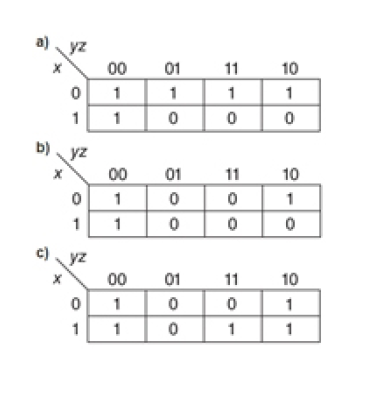Question
41 views

Write a simplified expression for the Boolean function defined by each of the following Kmaps.help_outlineImage TranscriptionclosezA 00 10 01 11 1 1 0 0 0 b) yz 01 00 11 10 x 1 0 0 1 0 0 0 1 1 c) yz 00 01 11 10 0 1 0 0 1 1 1 OO O fullscreen
check_circle

Step 1

K-map stands for Karnaugh map that provides a way of grouping the expression together on the basis of some common factors and eliminating those variables which are unwanted. For the given k- maps, Boolean expressions are formed using the concept of min terms (with result value 1) for sum of product. To find the Boolean expression of k map, all the 1’s are combined in a way to enclose maximum numbers of adjacent squares. Then, the expressions for the enclose terms are written. Here 1 denotes true and 0 denotes false.

Step 2

(a)

The given k map will form squares with maximum number of 1’s in it as shown below.

For each square, write the expression for the terms. The expression is formed as:

• For the square with two terms, the expression produced is Y’Z’.
• For the square enclosing four terms, the expression produced is X’.

The boolean expression for given K-map is X’+Y’Z’.

Step 3

(b)

The k map will form squares with maximum number of 1’s included in it as shown below.

For each square, write the expression for the terms. Here 1 denotes true and 0 denotes false. The expression is formed as:

• For the first square, the expression produced is Y’...

### Want to see the full answer?

See Solution

#### Want to see this answer and more?

Solutions are written by subject experts who are available 24/7. Questions are typically answered within 1 hour.*

See Solution
*Response times may vary by subject and question.
Tagged in

### Boolean Logic